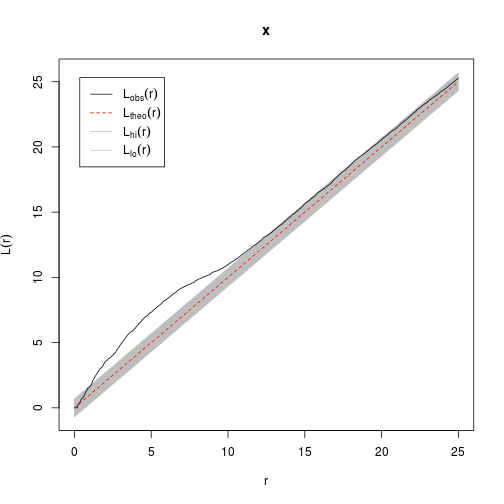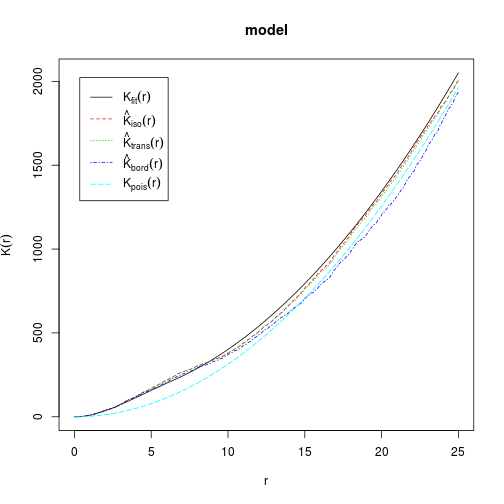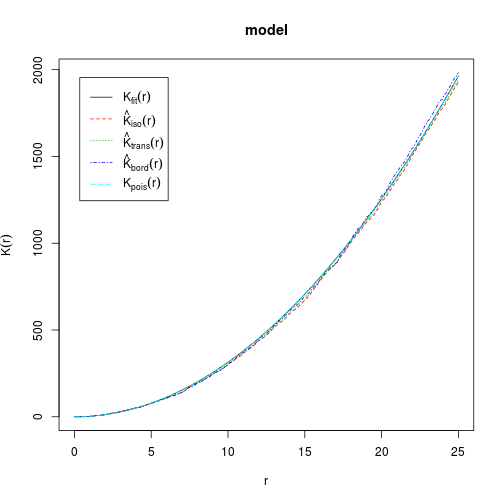# Fitting models of spatial clustering

Spatstat has functions for both simulating data from models that generate patterns and to fit models to the pattern.

You will need a lot of points in order to successfully fit models. The first step is to understand the logic behind the model you are going to fit.

## Matern cluster model

The Matern cluster model is one of the simplest to understand. It works like this. First of all the model supposes that the centre point (this is often called the “parent” in the literature) of each cluster falls randomly within the area. So the model for this is the same as the homogeneous poisson process.

Say our window is a 100 x 100 m plot.

``````library(spatstat)
``````
``````## Loading required package: mgcv
``````
``````## This is mgcv 1.7-24. For overview type 'help("mgcv-package")'.
``````
``````## Loading required package: deldir
``````
``````## deldir 0.0-22
``````
``````## spatstat 1.31-3 Type 'help(spatstat)' for an overview of spatstat
## licence information on polygon calculations
``````
``````set.seed(1)
win <- owin(c(0, 100), c(0, 100))

``````

The area therefore measures 10000 m2. So to simulate approximately 50 points within the area lamda should be 50/10000

``````pts <- rpoispp(50/10000, win = win)
plot(pts)
````````````pts
``````
``````##  planar point pattern: 45 points
## window: rectangle = [0, 100] x [0, 100] units
``````

OK, so that is a random pattern. How does the Matern model work. Well, think of doing this. Draw a circle around each point of radius r. Within this circle add some more points at random. If the circle is relatively small compared to the distance between the centres of the clusters then you will see clear clustering. If the radius is large then you won't. The number of points in each cluster is also random, but has a mean of mu. So if the radius around a simulated random set of parent points is 4m and there are on average 5 points per cluster we get a clear pattern and the quadrat test rejects CSR.

``````clust1 <- rMatClust(50/10000, r = 4, mu = 5, win = win)
plot(clust1)
````````````quadrat.test(clust1)
``````
``````##
##  Chi-squared test of CSR using quadrat counts
##
## data:  clust1
## X-squared = 71.27, df = 24, p-value = 2.805e-06
## alternative hypothesis: two.sided
##
## Quadrats: 5 by 5 grid of tiles
``````

However if the radius is large you won't notice any clustering any a quadrat test will not reject CSR.

``````clust2 <- rMatClust(50/10000, r = 40, mu = 5, win = win)
plot(clust2)
````````````quadrat.test(clust2)
``````
``````##
##  Chi-squared test of CSR using quadrat counts
##
## data:  clust2
## X-squared = 18.46, df = 24, p-value = 0.4396
## alternative hypothesis: two.sided
##
## Quadrats: 5 by 5 grid of tiles
``````

The nice thing about the Matern model is that it is simple to understand and interpret. You could think of the parent point literally, for example if your model consists of clusters of plants that grow around an initial coloniser. However there is no need to assume this. The “parent” could just represent the centre of an area into which a group of seeds disperse and survive.

## Testing using an envelope

If clustering occurs part at least of the L function will fall above the simulated envelope.

``````E <- envelope(clust1, Lest, nsim = 99, rank = 1, global = TRUE)
``````
``````## Generating 99 simulations of CSR  ...
## 1, 2, 3, 4, 5, 6, 7, 8, 9, 10, 11, 12, 13, 14, 15,
## 16, 17, 18, 19, 20, 21, 22, 23, 24, 25, 26, 27, 28, 29, 30,
## 31, 32, 33, 34, 35, 36, 37, 38, 39, 40, 41, 42, 43, 44, 45,
## 46, 47, 48, 49, 50, 51, 52, 53, 54, 55, 56, 57, 58, 59, 60,
## 61, 62, 63, 64, 65, 66, 67, 68, 69, 70, 71, 72, 73, 74, 75,
## 76, 77, 78, 79, 80, 81, 82, 83, 84, 85, 86, 87, 88, 89, 90,
## 91, 92, 93, 94, 95, 96, 97, 98,  99.
##
## Done.
``````
``````plot(E)
````````````##      lty col  key      label                                 meaning
## obs    1   1  obs  L[obs](r) observed value of L(r) for data pattern
## theo   2   2 theo L[theo](r)       theoretical value of L(r) for CSR
## hi     1   8   hi   L[hi](r)        upper critical boundary for L(r)
## lo     1   8   lo   L[lo](r)        lower critical boundary for L(r)
``````

The point at which the distance from the envelope is greatest should correspond to the radius used to generate the data.

## Fitting a model

It is possible to fit a model to the points. This will provide an estimate of the critical radius and the number of points per cluster. However you can't expect this to accurately find the underlying parameters as there is of course a random element. Unfortunately the fitting process does not provide confidence intervals for the model.

You also need to provide some starting values. It is important to give a good estimate of kappa (count the points and divide by the area)

``````model <- matclust.estK(clust1, startpar = c(kappa = 50/10000, R = 1))
plot(model)
````````````##        lty col    key            label
## fit      1   1    fit        K[fit](r)
## iso      2   2    iso   hat(K)[iso](r)
## trans    3   3  trans hat(K)[trans](r)
## border   4   4 border  hat(K)[bord](r)
## theo     5   5   theo       K[pois](r)
##                                               meaning
## fit    minimum contrast fit of Matern Cluster process
## iso      Ripley isotropic correction estimate of K(r)
## trans          translation-corrected estimate of K(r)
## border              border-corrected estimate of K(r)
## theo                         theoretical Poisson K(r)
``````
``````model
``````
``````## Minimum contrast fit (object of class "minconfit")
## Model: Matern Cluster process
## Fitted by matching theoretical K function to Kest(clust1)
## Parameters fitted by minimum contrast (\$par):
##  kappa      R
## 0.0114 3.3517
## Derived parameters of Matern Cluster process (\$modelpar):
##  kappa      R     mu
## 0.0114 3.3517 2.4468
## Converged successfully after 81 iterations
## Starting values of parameters:
## kappa     R
## 0.005 1.000
## Domain of integration: [ 0 , 25 ]
## Exponents: p= 2, q= 0.25
``````

Notice that the parameters are roughly around the size of those used to generate the pattern, but you can't realistically expect to find the same parameters due to the fact that three distinct random elements are involved (position of parent point, number of points per cluster and position of points around parent).

If you try to fit a model to the pattern that does not show clustering the paraemters will turn out to be vanishingly small.

``````model <- matclust.estK(clust2, startpar = c(kappa = 50/10000, R = 1))
plot(model)
````````````##        lty col    key            label
## fit      1   1    fit        K[fit](r)
## iso      2   2    iso   hat(K)[iso](r)
## trans    3   3  trans hat(K)[trans](r)
## border   4   4 border  hat(K)[bord](r)
## theo     5   5   theo       K[pois](r)
##                                               meaning
## fit    minimum contrast fit of Matern Cluster process
## iso      Ripley isotropic correction estimate of K(r)
## trans          translation-corrected estimate of K(r)
## border              border-corrected estimate of K(r)
## theo                         theoretical Poisson K(r)
``````
``````model
``````
``````## Minimum contrast fit (object of class "minconfit")
## Model: Matern Cluster process
## Fitted by matching theoretical K function to Kest(clust2)
## Parameters fitted by minimum contrast (\$par):
##  kappa      R
## 1751.2  325.6
## Derived parameters of Matern Cluster process (\$modelpar):
##     kappa         R        mu
## 1.751e+03 3.256e+02 1.319e-05
## Converged successfully after 39 iterations
## Starting values of parameters:
## kappa     R
## 0.005 1.000
## Domain of integration: [ 0 , 25 ]
## Exponents: p= 2, q= 0.25
``````